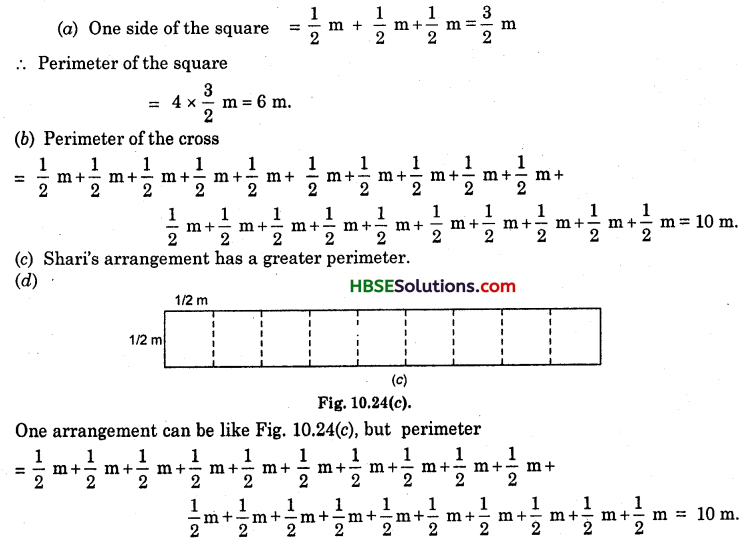# HBSE 6th Class Maths Solutions Chapter 10 Mensuration Ex 10.1

Haryana State Board HBSE 6th Class Maths Solutions Chapter 10 Mensuration Ex 10.1 Textbook Exercise Questions and Answers.

## Haryana Board 6th Class Maths Solutions Chapter 10 Mensuration Exercise 10.1

Question 1.
Find the perimeter of each of the following figures :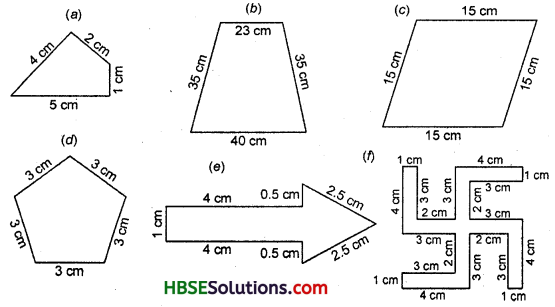Solution:
(a) Perimeter = 5 cm + 1 cm + 2 cm + 4 cm = 12 cm.
(b) Perimeter = 40 cm + 35 cm + 23 cm + 35 cm = 133 cm.
(c) Perimeter = 15 cm +15 cm + 15 cm + 15 cm = 60 cm.
(d) Perimeter = 3 cm + 3 cm + 3 cm + 3 cm + 3 cm = 15 cm.
(e) Perimeter = 1 cm + 4 cm + 0.5 cm + 2.5 cm + 2.5 cm + 0.5 cm + 4 cm = 15 cm.
(f) Perimeter = 4 cm + 1 cm + 3 cm + 2 cm + 3 cm + 4 cm + 1 cm + 3 cm + 2 cm + 3 cm + 4 cm + 1 cm + 3 cm + 2 cm + 3 cm + 4 cm + 1 cm + 3 cm + 2 cm + 3 cm
= 52 cm.Question 2.
The lid of a rectangular box of sides 40 cm by 10 cm is sealed all around with tape. What is the length of the tape required ?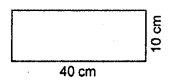Solution:
Length of the rectangular box (l) = 40 cm
Breadth of the rectangular box (b) = 10 cm
Perimeter of the rectangular box
= 2 x (l + b)
= 2 x (40 + 10) cm
= 2 x 50 = 100 cm
Hence, length of the tape required = 100 cm or 1m.

Question 3.
A table-top measures 2 m 25 cm by 1 m 50 cm. What is the perimeter of the top of the table ?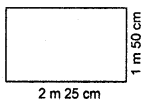Solution:
Length of the table-top (l)
= 2 m 25 cm
= 2.25 m
Breadth of the table-top (b)
= 1 m 50 cm
= 1.50 m
Perimeter of the table-top
= 2 x (l + b)
= 2 x (2.25 + 1.50) m
= 2 x 3.75
= 7.50 m.

Question 4.
What is the length of the wooden strip required to frame a photograph of length and breadth 32 cm and 21 cm respectively ?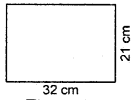Solution:
Length of the frame (l)
= 32 cm
Breadth of the frame (b)
= 21 cm
Perimeter of the frame
= 2 x (l + b)
= 2 x (32 cm + 21 cm)
= 2 x 53 cm
= 106 cm.
Hence, length of the wooden strip required to frame a photograph = 106 cm.Question 5.
A rectangular piece of land measures 0.7 km by 0.5 km. Each side is to be fenced with 4 rows of wires. What is the length of wire needed ?
Solution:
Length (l) = 0.7 km
Breadth (b) = 0.5 km
Perimeter – 2 x (l + b)
= 2 x (0.7 + 0.5) km
= 2 x 1.2 = 2.4 km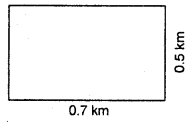Length of wire needed to fence with 4 rows = 4 x 2.4 = 9.6 km.

Question 6.
Find the perimeter of each of the following shapes :
(a) A triangle of sides 3 cm, 4 cm and 5 cm.
(b) An equilateral triangle of side 9 cm.
(c) An isosceles triangle with equal sides 8 cm each and third side 6 cm.
Solution:
(a) Perimeter of the triangle
= 3 cm + 4 cm + 5 cm = 12 cm.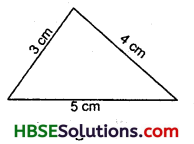(b) One side of an equilateral
Δ = 9 cm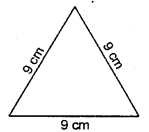.’. Perimeter of the triangle
= 3 x length of one side
= 3 x 9 cm = 27 cm.

(c) Perimeter of isosceles triangle
= 8 cm + 8 cm + 6 cm
= 22 cm.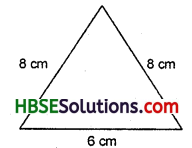Question 7.
Find the peri-meter of a triangle with sides measuring 10 cm, 14 cm and 15 cm.
Solution:
Perimeter of the triangle
= 10 cm + 14 cm + 15 cm
= 39 cm.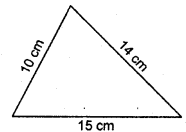Question 8.
Find the perimeter of a regular hexagon with each side measuring 8 m.
Solution:
One side of a regular hexagon = 8 mPerimeter of regular hexagon = 6 x one side
= 6 x 8 m
= 48 m.

Question 9.
Find the side of a square whose perimeter is 20 m.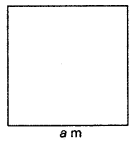Solution:
Let, one side of a square = am
Perimeter of the square = 20 m
4 x a = 20
a = $$\frac{20}{4}$$ = 5 cm
Hence, one side of the square = 5 m.Question 10.
The perimeter of a regular pentagon is 100 cm. How long is each side?
Solution:
Let one side of the regular pentagon = a cm
Perimeter of the regular pentagon = 100 cm
5 x a = 100
=> a = $$\frac{100}{5}$$ = 20 cm.
Hence, each side of a regular pentagon = 20 cm.

Question 11.
A piece of string is 30 cm long. What will be the length of each side if the string is used to form :
(a) a square ?
(b) an equilateral triangle ?
(c) a regular hexagon ?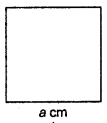Solution:
(a) Let one side of a square = a cm
Perimeter of the square = 30 cm
4 x a = 30 cm
=> a = $$\frac{30}{4}$$ cm = 7.5 cm
Hence, length of each side of the square = 7.5 cm.

(b) Let each side of the equilateral Δ = a cm
Perimeter of the equilateral Δ = 30 cm
3 x a = 30 cm
a = $$\frac{30}{3}$$
= 10 cm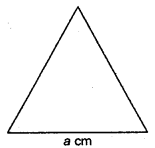Hence, each side of the equilateral Δ = 10 cm.

(c) Let each side of the regular hexagon
= a cm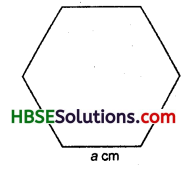Perimeter of the regular hexagon = 30 cm
6 x a = 30 cm
=> a = $$\frac{30}{6}$$ = 5 cm

Question 12.
Two sides of a triangle are 12 cm and 14 cm. The perimeter of the triangle is 36 cm. What is the third side ?
Solution:
Let the third side of a triangle = x cm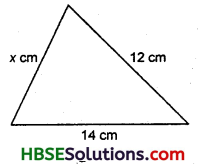Perimeter of the triangle = 36 cm
.-. 14 cm + 12 cm + x cm = 36 cm
=> 26 cm + x cm = 36 cm
x = 36 – 26 = 10 cm
Hence, the third side of the triangle = 10 cm.Question 13.
Find the cost of fencing a square park of side 250 m at the rate of Rs. 20 per metre.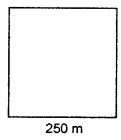Solution:
Each side of the square park = 250 m
Perimeter of the square park
= 4 x 250 m
= 1000 m
Cost of one metre of wire = Rs. 20
Cost of fencing the square park = Rs. 20 x 1000
= Rs. 20,000.

Question 14.
Find the cost of fencing a rectangular park of length 175 m and breadth 125 m at the rate of Rs. 12 per metre.
Solution:
Length of the rectangular park (l) = 175 m
Breadth of the rectangular park (b) = 125 m
Perimeter of the rectangular park . = 2 x (l + b)
= 2 x (175 + 125) m
= 2 x 300
= 600 m
Cost of one metre of wire = Rs. 12
.•. Cost of fencing the rectangular park
= Rs. 12 x 600
= Rs. 7200.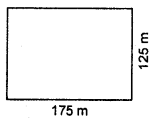Question 15.
Sweety runs around a square park of side 75 m. Bulbul runs around a rectangle with length 60 metre and breadth 45 metre. Who covers less distance ?
Solution: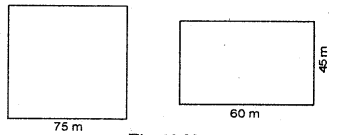Each side of the square park = 75 m
Perimeter of the square park = 4 x 75 = 300 m
.’. Distance covered by Sweety = 300 m
Now, length of the rectangular park (l)
= 60 m
breadth of the rectangular park (b) = 45 m
Perimeter of the rectangular park = 2 x (l+ b)
= 2 x (60 + 45)
= 2 x 105 = 210 m
Distance covered by Bulbul = 210 m
Hence, Bulbul covers less distance.

Question 16.
What is the perimeter of each of the following figures ? What do you infer from the answers ?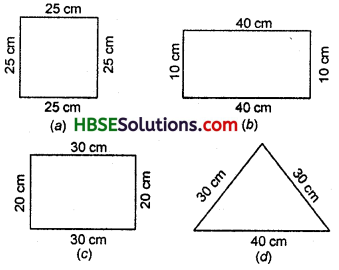Solution:
(a) One side of the square = 25 cm
Perimeter of the square
=4 x 25 = 100 cm.

(b) Length of the rectangle (l) = 40 cm
Breadth of the rectangle (b) = 10 cm
Perimeter of the rectangle = 2 x (l + b)
= 2 x (40 + 10) cm
= 2 x 50 = 100 cm.

(c) Length of the rectangle (l) = 30 cm
Breadth of the rectangle (b) = 20 cm
.’. Perimeter of the rectangle
= 2 x (l + b)
= 2 x (30 + 20) cm
= 2 x 50 = 100 cm.(d) Sides of the triangle are 40 cm, 30 cm and 30 cm.
Perimeter of the triangle
= 40 cm + 30 cm + 30 cm
= 100 cm.
We infer from the answers that all the figures have the same perimeter.

Question 17.
Avneet buys 9 square paving slabs, each of a side of 1/2 m. He lays them in the form of a square.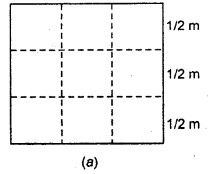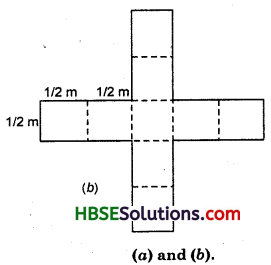(a) What is the perameter of his arrangement [Fig. 10.24(a)]?
(b) Shari does not like his arrangement.
She gets him to lay them out like a cross [Fig. 10.24(b)]. What is the perimeter of her arrangement ?
(c) Which has a greater perimeter ?
(d) Avneet wonders if there is a way of getting an even greater perimeter. Can you find a way of doing this ? (The paving slabs must meet along complete edges, they cannot be broken).
Solution: